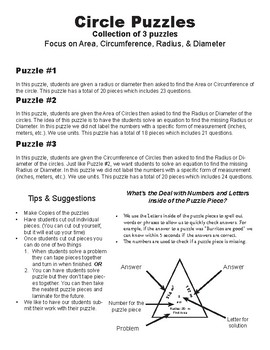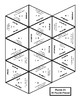# Circle Puzzles (Collection of 3)Subject
Resource Type
File Type

PDF

(242 KB|7 pages)
Product Description

This is a collection of 3 puzzles that focuses on Area, Circumference, Radius, & Diameter of Circles. Students cut out the different puzzle pieces and then solve the problems. When a student has solved a problem they match the side with the problem with the side that has the solution.

Each puzzle has between 18 to 20 puzzle pieces (shaped like triangles) with a range of 21 to 24 questions.

In the first puzzle, students are given the radius or diameter then asked to find the Area or Circumference of that circle.

The second puzzle gives students the Area of a Circle. Students when then be required to find Radius or the Diameter.

The third puzzle gives each student the Circumference and the students must find the Radius or Circumference.

This puzzle is ideal for:

1) Review

2) Stations

3) Intervention

4) Challenge work for students that have completed an assignment

5) Activity for Subs to use

6) A change of pace from traditional worksheets

Total Pages
7 pages
Included
Teaching Duration
3 days
Report this Resource to TpT
Reported resources will be reviewed by our team. Report this resource to let us know if this resource violates TpT’s content guidelines.